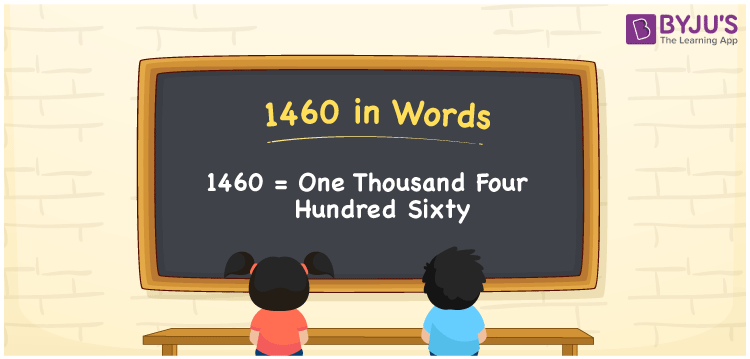# 1460 in Words

In English words, 1460 represents “One Thousand Four Hundred Sixty”. The number 1460 is a cardinal number, which helps to count the objects, and so on. For example, there are 1460 roses in a shop. In this article, let us discuss how to write the number 1460 in words using the place value system with a complete explanation.

 1460 in Words: One Thousand Four Hundred Sixty. One Thousand Four Hundred Sixty in Numerical Form: 1460.

## 1460 in English Words## How to Write 1460 in Words?

The following table helps you to understand the place values of the number 1460.

 Thousands Hundreds Tens Ones 1 4 6 0

The expanded form of 1460 is as follows:

= 1 × Thousand + 4 × Hundred + 6 × Ten + 0 × One

= 1 × 1000 + 4 × 100 + 6 × 10

= 1000 + 400 + 60

= 1460

= one thousand four hundred sixty

Hence, 1460 in words is One thousand four hundred sixty.

The number 1460 lies between 1459 and 1461.

1460 in words – One thousand four hundred sixty

Is 1460 an odd number? – No

Is 1460 an even number? – Yes

Is 1460 a perfect square number? – No

Is 1460 a perfect cube number? – No

Is 1460 a prime number? – No

Is 1460 a composite number? – Yes

## Frequently Asked Questions on 1460 in Words

### How to spell 1460 in English words?

1460 in words is one thousand four hundred sixty.

### Simplify 1000 + 460, and express it in words.

Simplifying 1000 + 460, we get 1460. Hence, 1460 in words is one thousand four hundred sixty.

### Is 1460 a prime number?

No, 1460 is not a prime number.